JOINProblem Statement
Contest: Marathon Match 85
Problem: CollageMaker

### Problem Statement

IMPORTANT: This problem is used for two simultaneous matches: TCO'14 Marathon Round 3 and Marathon Match 85. You can compete in TCO'14 Marathon Round 3 only if you are eligible for TCO'14 and haven't advanced to TCO'14 Marathon Championship Round. You can compete in Marathon Match 85 if you can't compete in TCO'14 Marathon Round 3 or would like to skip it by some reason. Competing in both matches is not allowed. Doing so will lead to disqualification. Note that registration does not count as competing. In order to be considered a competitor, you need to make at least one submit (example or full).

In this problem, you will be given a larger target image and 200 smaller source images. All images are gray-scale. The goal is to create a collage from the source images that looks as similar to the target image as possible.

For each source image, you can ignore or use it. If you use the image, the following steps are made:

1. Suppose that original image dimensions are HSxWS.
2. First, new image dimensions hsxws (1 <= hs <= HS, 1 <= ws <= WS) are chosen.
3. Then, then image is downscaled to the chosen dimensions (exact method used is described below).
4. Finally, the image is located somewhere within the target image.

Note that source images can't be rotated, flipped or upscaled by any dimension. The resulted placement of source images must be such that each pixel of the target image is covered by exactly one source image.

Downscaling of each source image is to be performed as follows:

• The original HSxWS image is upscaled to become (HS*hs)x(WS*ws). To achieve that, each pixel is replaced with hsxws solid rectangle with the same color.
• Given r and c, 0 <= r < hs, 0 <= c < ws, consider rectangle within the upscaled (HS*hs)x(WS*ws) image that contains rows from r*HS to (r+1)*HS-1, inclusive, and columns from c*WS to (c+1)*WS-1, inclusive.
• The color of pixel at row r, column c within the downscaled hsxws image is set to the arithmetic average of pixel intensities within this rectangle rounded towards the nearest integer (by standard mathematical rounding rules; for example, 3.4 is rounded to 3 and 3.5 or 3.6 are rounded to 4).

Test data

All test cases in this problem use the same dataset of 1,000 images that can be downloaded here. Folder "300px" contains possible target images and folder "100px" contains possible source images. In fact, both folders contain the same 1,000 images, but images in "100px" folder are smaller. Each image was chosen as a random file from Wikipedia (using this query) that has a MIME type of "image/jpeg" and both width and height between 2,500 and 5,000, inclusive. After being chosen, the image is converted to gray-scale, downscaled so that the maximum of its dimensions is 300 pixels and saved to "300px" folder. Then, the same image is downscaled so that the maximum of its dimensions is 100 pixels and saved to "100px" folder. No manual image screening was performed.

Each test case is generated using the same algorithm. The difference between test cases is only in seed value which is used to initialize a pseudorandom generator. The target image is always chosen as image from "300px" folder with number seed%1000. The source images are selected as 200 random images from "100px" folder with distinct numbers (each of them being not equal to seed%1000).

Implementation

You will need to implement the method compose. Its input parameter data is an array/vector/tuple of integers that should be interpreted as consecutive stream of integers which contains 201 image descriptions. First image description corresponds to the target image and the next 200 image descriptions are source images. Each image descriptions starts from two integers H (image height, in pixels) and W (image width, in pixels). It is followed by H*W integers where (r*W+C)-th integer (0-based) describes the pixel intensity at row r, column c (both 0-based). Each intensity is between 0 and 255, inclusive.

The return value must be an array/vector/tuple containing exactly 800 integers. First 4 integers should describe your decision for first source image, next 4 integers for the second source image and so on. If you would like to ignore a particular image, then all 4 integers must be equal to -1. Otherwise, the integers must be (in order):

• The top row (0-based) within the target image which should be covered by the given source image.
• The leftmost column (0-based) within the target image which should be covered by the given source image.
• The bottom row (0-based) within the target image which should be covered by the given source image.
• The rightmost column (0-based) within the target image which should be covered by the given source image.

Scoring

For each test case we will calculate your raw and normalized scores. If you were not able to produce a valid return value, then your raw score is -1 and the normalized score is 0. Otherwise, the raw score is calculated as follows. Let target(r, c) and collage(r, c) be the intensity of pixel at row r, column c within the target image and your collage image (the union of all source images you used), respectively. Let also HT and WT be the height and the width of the target image, in pixels. Then your raw score is equal to SquareRoot(S / (HT * WT)) (exact division), where S is the sum of (target(r, c) - collage(r, c))^2 over all pixels. The normalized score for each test is 1,000,000.0 * BEST / YOUR, where BEST is the lowest non-negative raw score currently obtained on this test case (considering only the last submission from each competitor). Finally, your total score is equal to the arithmetic average of normalized scores on all test cases.

You can see your raw scores on each example test case by making an example submit. You can also see total scores of all competitors on provisional test set in the match standings. No other information about scores is available during the match.

Tools

An offline tester/visualizer is available. You can use it to test/debug your solution locally. You can also check its source code for exact implementation of test case generation and score calculation. In particular, the code that performs source images downscaling is between lines 67 and 130, inclusive.

### Definition

 Class: CollageMaker Method: compose Parameters: int[] Returns: int[] Method signature: int[] compose(int[] data) (be sure your method is public)

### Notes

-The time limit is 10 seconds per test case (this includes only the time spent in your code). The memory limit is 1024 megabytes.
-The code size limit is 256 kilobytes.
-The compilation time limit is 60 seconds. You can find information about compilers that we use and compilation options here.
-There are 10 example test cases and 100 full submission (provisional) test cases. All provisional test cases have pairwise distinct values of seed%1000.
-There will be at least 1000 system test cases and the number of system test cases will be a multiple of 1000. Each seed%1000 value will occur within the same number of system test cases.

### Examples

0)

`seed = 1`
 Target image:Source images (each downscaled to 30x30):1)

`seed = 2`
 Target image:Source images (each downscaled to 30x30):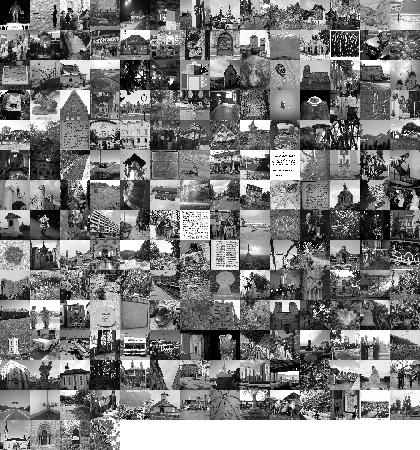2)

`seed = 3`
 Target image: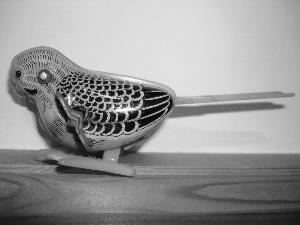Source images (each downscaled to 30x30):3)

`seed = 4`
 Target image:Source images (each downscaled to 30x30):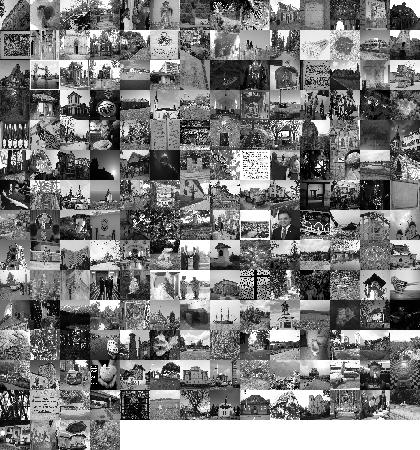4)

`seed = 5`
 Target image:Source images (each downscaled to 30x30):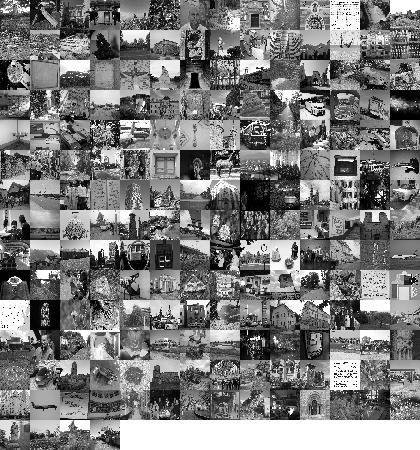5)

`seed = 6`
 Target image: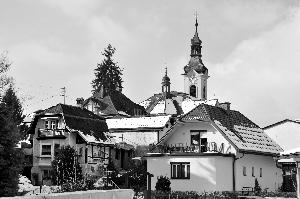Source images (each downscaled to 30x30):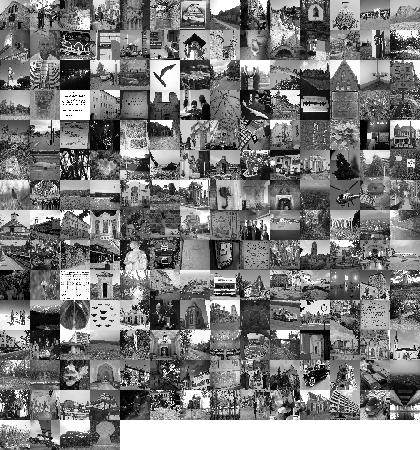6)

`seed = 7`
 Target image: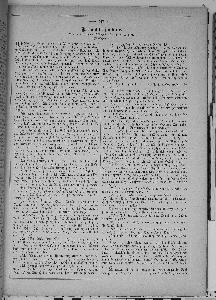Source images (each downscaled to 30x30):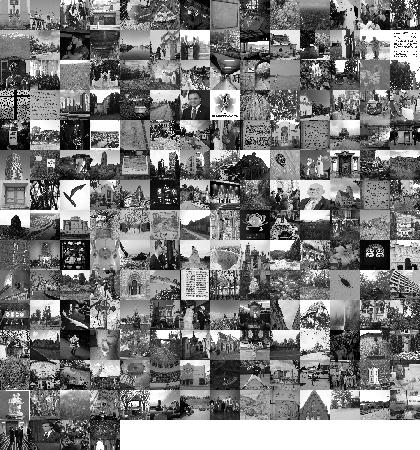7)

`seed = 8`
 Target image: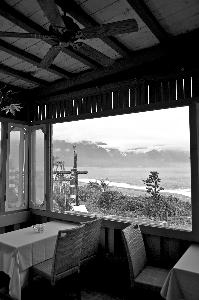Source images (each downscaled to 30x30):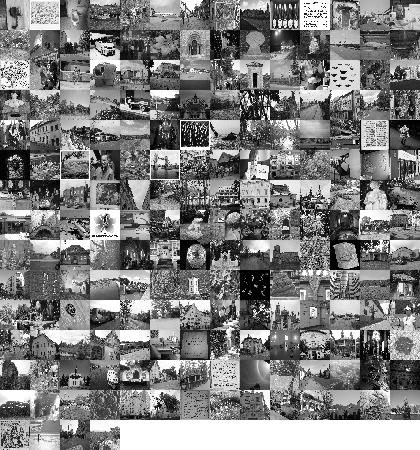8)

`seed = 9`
 Target image:Source images (each downscaled to 30x30):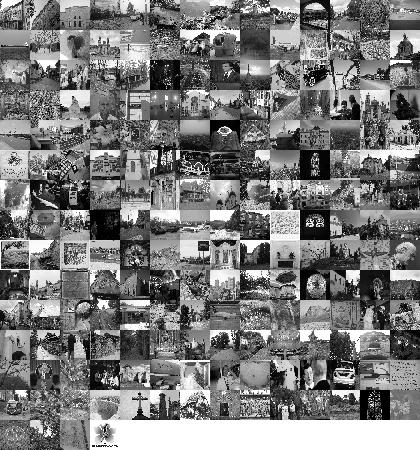9)

`seed = 10`
 Target image:Source images (each downscaled to 30x30):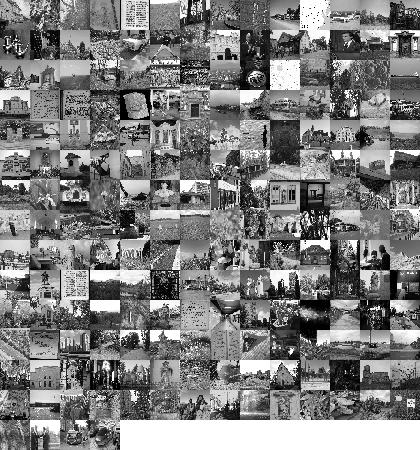This problem statement is the exclusive and proprietary property of TopCoder, Inc. Any unauthorized use or reproduction of this information without the prior written consent of TopCoder, Inc. is strictly prohibited. (c)2010, TopCoder, Inc. All rights reserved.